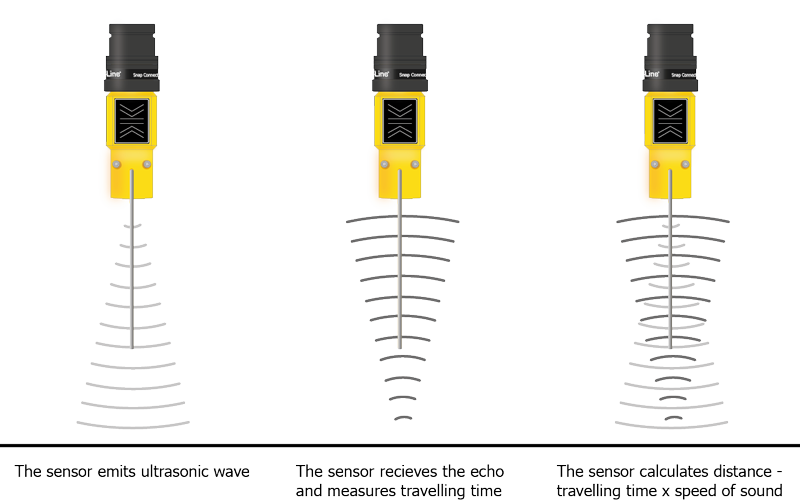# What is ultrasound and how does an ultrasonic grade sensor work?

Lars Boss Lindholm / 02-12-2015Today, modern grade sensors used in asphalt paving for levelling control are based on ultrasound. The advantage of a non-contact sensor removed from the sticky asphalt material is obvious, but how exactly does an ultrasonic grade sensor work?

### What is ultrasound?

Ultrasound is basically normal sound waves with frequencies so high that they are inaudible to the human ear. Ultrasound can be used to measure distances to objects by measuring the delay between the transmission of an ultrasound pulse and the return of the echo. This is the well-known way bats navigate in the dark, and the same principles your ultrasonic grade sensor applies to measure the distance to a reference such as a string, a curb or the ground.

### How does an ultrasonic grade sensor work?

Ultrasonic grade sensors are equipped with an ultrasonic transducer that both generates pulses and receives the returning echoes of each pulse. When the ultrasonic waves emitted by the sensor meet the physical reference, they bounce back as an echo, which can be detected by the sensor. The time between a pulse is sent out and its echo is received is called the travelling time. Because we know the speed of sound, the sensor can use the measured travelling time to determine the distance to the reference.

It does that just like you would determine the travelled distance in a car by multiplying the time behind the wheel with the speed of the car. For example, we know that

1 hour driving  x 10 miles per hour = 10 miles distance travelled
Driving  time   x       speed             =    distance

For the ultrasonic sensor, that means

Travelling time x speed of sound = distance to reference

When positioned on the screed on an asphalt paver, the grade sensor is able to maintain the desired asphalt thickness paved by ensuring that the distance to the reference remains the same. It does that by constantly checking the travelling time and calculating the distance to the reference to make sure it remains the same.

## g221-us-beam-tf-technologies_800x500.pngWhen a sensor is mounted to a paver and the screed starts to rise, the sensor will instantly detect an increase in travelling time and calculate the new increased distance to the reference. This prompts a message to the controller to start regulation of the tow point downwards, to bring the grade sensor back to its original distance above the reference to maintain the mat thickness.

This regulation happen instantaneously as the sensor is able to detect the change in travelling time much sooner than the naked eye can spot an increase in mat thickness.  That is why the grade sensor is able to very precisely copy the reference, far beyond what is humanly possible, and place a mat at the required depth with millimeter precision.

### Why do grade sensors need a reference bail?

As we just learned, when the travelling time and the speed of sound are known, then the sensor can determine the distance to the reference. The only challenge is that the speed of sound changes with different ambient temperatures. This poses a real challenge in asphalt paving, where temperature shifts can be huge due to factors such as changing wind directions and radiant heat.

As the grade sensor needs to know the current speed of sound to calculate the correct distance at all times, the grade sensor must overcome this obstacle and compensate for variations in temperature. It does that with a reference bail. The reference bail is used by the grade sensor to figure out the speed of sound by providing the sensor with a known distance from which the sensor can calculate the current speed of sound, based on the same formula used to calculate the distance to the reference. Brushing up on our math skills, we know that

Travelling time x speed = distance

can be rewritten to

Distance/travelling time = speed

Since the distance to the reference bail is known by the grade sensor, and it is able to measure the travelling time between the sensor and the reference bail, the sensor can calculate the speed of sound.

So, when the transducer sends out a pulse it actually receives two echoes: one from the reference bail and one from the physical reference. The first echo is used to calculate the current speed of sound and the second echo is used to determine the accurate distance to the reference.

In this way you can measure the accurate distance to the reference in various ambient temperatures, when you use a sonic sensor with a reference bail.

Back to list